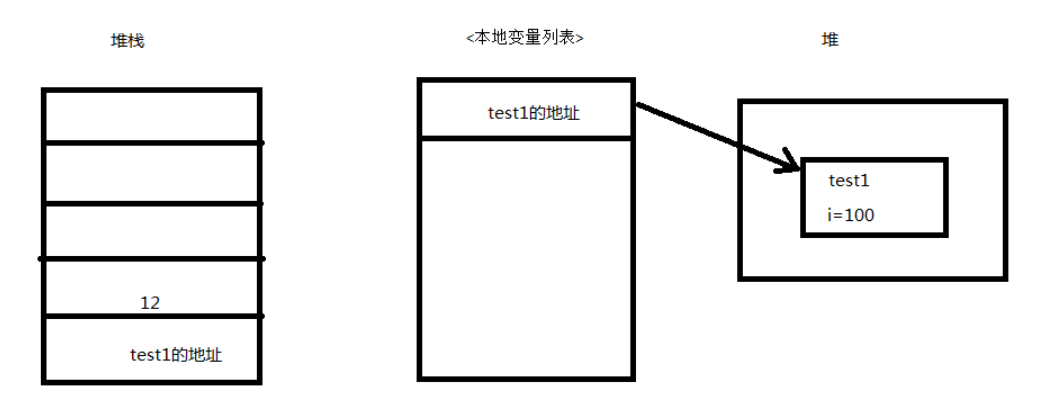+关注继续查看

# 探讨贡献提名典礼# （可能也许大概差不离就是）最终版代码（也可能不是）

/// <summary>
/// 判断是否为数值类型。
/// </summary>
/// <param name="t">要判断的类型</param>
/// <returns>是否为数值类型</returns>
public static bool IsNumericType(this Type t)
{
var tc = Type.GetTypeCode(t);
return (t.IsPrimitive && t.IsValueType && !t.IsEnum && tc != TypeCode.Char && tc != TypeCode.Boolean) || tc == TypeCode.Decimal;
}

/// <summary>
/// 判断是否为可空数值类型。
/// </summary>
/// <param name="t">要判断的类型</param>
/// <returns>是否为可空数值类型</returns>
public static bool IsNumericOrNullableNumericType(this Type t)
{
return t.IsNumericType() || (t.IsNullableType() && t.GetGenericArguments().IsNumericType());
}

/// <summary>
/// 判断是否为可空类型。
/// 注意，直接调用可空对象的.GetType()方法返回的会是其泛型值的实际类型，用其进行此判断肯定返回false。
/// </summary>
/// <param name="t">要判断的类型</param>
/// <returns>是否为可空类型</returns>
public static bool IsNullableType(this Type t)
{
return t.IsGenericType && t.GetGenericTypeDefinition() == typeof(Nullable<>);
}

# 为了累死电脑而设计的测试用代码

[TestClass]
public class BasicTest
{
[TestMethod]
public void 数值类型判断测试()
{
for (int i = 0; i < 500000; i++)
{
Assert.IsTrue((591).GetType().IsNumericType());
Assert.IsTrue((31.131).GetType().IsNumericType());
Assert.IsTrue((31.131f).GetType().IsNumericType());
Assert.IsTrue(((Int64)31).GetType().IsNumericType());
Assert.IsTrue((new decimal(31.351)).GetType().IsNumericType());
Assert.IsTrue((new Decimal(31.351)).GetType().IsNumericType());
Assert.IsTrue(((byte)31).GetType().IsNumericType());
Assert.IsTrue(((UInt64)31).GetType().IsNumericType());
Assert.IsTrue(((UIntPtr)31).GetType().IsNumericType());
Assert.IsTrue(((short)31).GetType().IsNumericType());
Assert.IsTrue(((Single)31).GetType().IsNumericType());

Assert.IsTrue((typeof(Int64?)).IsNumericOrNullableNumericType());
Assert.IsTrue((typeof(UInt64?)).IsNumericOrNullableNumericType());
Assert.IsTrue((typeof(decimal?)).IsNumericOrNullableNumericType());
Assert.IsTrue((typeof(Decimal?)).IsNumericOrNullableNumericType());
Assert.IsTrue((typeof(UIntPtr?)).IsNumericOrNullableNumericType());
Assert.IsTrue((typeof(byte?)).IsNumericOrNullableNumericType());
Assert.IsTrue((typeof(Single?)).IsNumericOrNullableNumericType());
Assert.IsTrue((typeof(Double?)).IsNumericOrNullableNumericType());
Assert.IsTrue((typeof(float?)).IsNumericOrNullableNumericType());
Assert.IsTrue((typeof(double?)).IsNumericOrNullableNumericType());
Assert.IsTrue((typeof(int?)).IsNumericOrNullableNumericType());
Assert.IsTrue((typeof(short?)).IsNumericOrNullableNumericType());
Assert.IsTrue((typeof(Nullable<Byte>)).IsNumericOrNullableNumericType());

Assert.IsFalse(DateTime.Now.GetType().IsNumericType());
Assert.IsFalse(TimeSpan.FromDays(2).GetType().IsNumericType());
Assert.IsFalse("aacc".GetType().IsNumericType());
Assert.IsFalse(System.UriPartial.Path.GetType().IsNumericType());
Assert.IsFalse('c'.GetType().IsNumericType());
Assert.IsFalse(false.GetType().IsNumericType());

Assert.IsFalse((typeof(DateTime?)).IsNumericOrNullableNumericType());
Assert.IsFalse((typeof(Char?)).IsNumericOrNullableNumericType());
Assert.IsFalse((typeof(char?)).IsNumericOrNullableNumericType());
Assert.IsFalse((typeof(System.UriPartial?)).IsNumericOrNullableNumericType());
Assert.IsFalse((typeof(Boolean?)).IsNumericOrNullableNumericType());
Assert.IsFalse((typeof(bool?)).IsNumericOrNullableNumericType());
}

}
}

# 老外！不服跑个分？JEFFERY YOU 提出应该做一个测试，确实数据最有说服力。return t == typeof(int)
|| t == typeof(double)
|| t == typeof(long)
|| t == typeof(short)
|| t == typeof(float)
|| t == typeof(Int16)
|| t == typeof(Int32)
|| t == typeof(Int64)
|| t == typeof(uint)
|| t == typeof(UInt16)
|| t == typeof(UInt32)
|| t == typeof(UInt64)
|| t == typeof(sbyte)
|| t == typeof(Single)
|| t == typeof(Decimal)
|| t == typeof(Byte)
|| t == typeof(UIntPtr)
|| t == typeof(IntPtr);return t == typeof(Int16)
|| t == typeof(Int32)
|| t == typeof(Int64)
|| t == typeof(Single)
|| t == typeof(Double)
|| t == typeof(UInt16)
|| t == typeof(UInt32)
|| t == typeof(UInt64)
|| t == typeof(Byte)
|| t == typeof(Decimal)
|| t == typeof(SByte)
|| t == typeof(UIntPtr)
|| t == typeof(IntPtr);TypeCode 枚举中是没有  IntPtr 和 UIntPtr 项的，所以还是要做额外判断。

if (t.IsEnum) return false;
var tc = Type.GetTypeCode(t);
switch (tc)
{
case TypeCode.Int16:
case TypeCode.Int32:
case TypeCode.Int64:
case TypeCode.Single:
case TypeCode.Double:
case TypeCode.UInt16:
case TypeCode.UInt32:
case TypeCode.UInt64:
case TypeCode.Byte:
case TypeCode.Decimal:
case TypeCode.SByte:
return true;
default:
return t == typeof(UIntPtr) || t == typeof(IntPtr);
}# 巡回总结报告会演讲

## （微软：卧槽，看你们这么苦逼，我给你们直接做一个属性出来吧，请期待.Net 框架v10.29博主生日特别无码汉化激情未删减导演剪辑泄露蓝光3D版………嗯，我们将其委托给暴雪工作室开发。）【.Net底层剖析】2.stfld指令-给对象的字段赋值
【.Net底层剖析】2.stfld指令-给对象的字段赋值
57 0.NET对象清理
.NET对象清理
53 0​## 如何优雅地使用腾讯云COS-.NET篇 代码下载地址 https://github.com/whuanle/txypx20190809 前提 创建子账号 打开 https://console.cloud.tencent.com/cam 创建子用户，设置子账号策略为 AdministratorAccess ，或者参考https://cloud.tencent.com/document/product/436/11714 ，添加访问 COS 的权限 记录子用户的 账号ID。
954 0.NET读取json数据并绑定到对象

765 01493 01728 01493 0.Net Web Api返回Json数据中原对象变量名大小写问题

2075 0.NET[C#]中实现实体对象深拷贝(克隆/复制)的几种方法
.NET[C#]中实现实体对象深拷贝(克隆/复制)的几种方法，总有一种适合你。 方式一 使用二进制流 using System; using System.IO; using System.Runtime.
3426 0.NET平台开源项目速览(14)最快的对象映射组件Tiny Mapper
1322 0

Gululu重新定义儿童饮水习惯